Visualize the distribution of the simulation-based inferential statistics or the theoretical distribution (or both!).

Learn more in vignette("infer").

visualize(data, bins = 15, method = "simulation", dens_color = "black", ...)

visualise(data, bins = 15, method = "simulation", dens_color = "black", ...)

## Arguments

data A distribution. For simulation-based inference, a data frame containing a distribution of calculate()d statistics or fit()ted coefficient estimates. This object should have been passed to generate() before being supplied or calculate() to fit(). For theory-based inference, the output of assume(). The number of bins in the histogram. A string giving the method to display. Options are "simulation", "theoretical", or "both" with "both" corresponding to "simulation" and "theoretical". If data is the output of assume(), this argument will be ignored and default to "theoretical". A character or hex string specifying the color of the theoretical density curve. Other arguments passed along to \ggplot2\ functions.

## Value

For calculate()-based workflows, a ggplot showing the simulation-based distribution as a histogram or bar graph. Can also be used to display theoretical distributions.

For assume()-based workflows, a ggplot showing the theoretical distribution.

For fit()-based workflows, a patchwork object showing the simulation-based distributions as a histogram or bar graph. The interface to adjust plot options and themes is a bit different for patchwork plots than ggplot2 plots. The examples highlight the biggest differences here, but see patchwork::plot_annotation() and patchwork::&.gg for more details.

## Details

In order to make the visualization workflow more straightforward and explicit, visualize() now only should be used to plot distributions of statistics directly. A number of arguments related to shading p-values and confidence intervals are now deprecated in visualize() and should now be passed to shade_p_value() and shade_confidence_interval(), respectively. visualize() will raise a warning if deprecated arguments are supplied.

shade_p_value(), shade_confidence_interval().

## Examples


# generate a null distribution
null_dist <- gss %>%
# we're interested in the number of hours worked per week
specify(response = hours) %>%
# hypothesizing that the mean is 40
hypothesize(null = "point", mu = 40) %>%
# generating data points for a null distribution
generate(reps = 1000, type = "bootstrap") %>%
# calculating a distribution of means
calculate(stat = "mean")

# or a bootstrap distribution, omitting the hypothesize() step,
# for use in confidence intervals
boot_dist <- gss %>%
specify(response = hours) %>%
generate(reps = 1000, type = "bootstrap") %>%
calculate(stat = "mean")

# we can easily plot the null distribution by piping into visualize
null_dist %>%
visualize()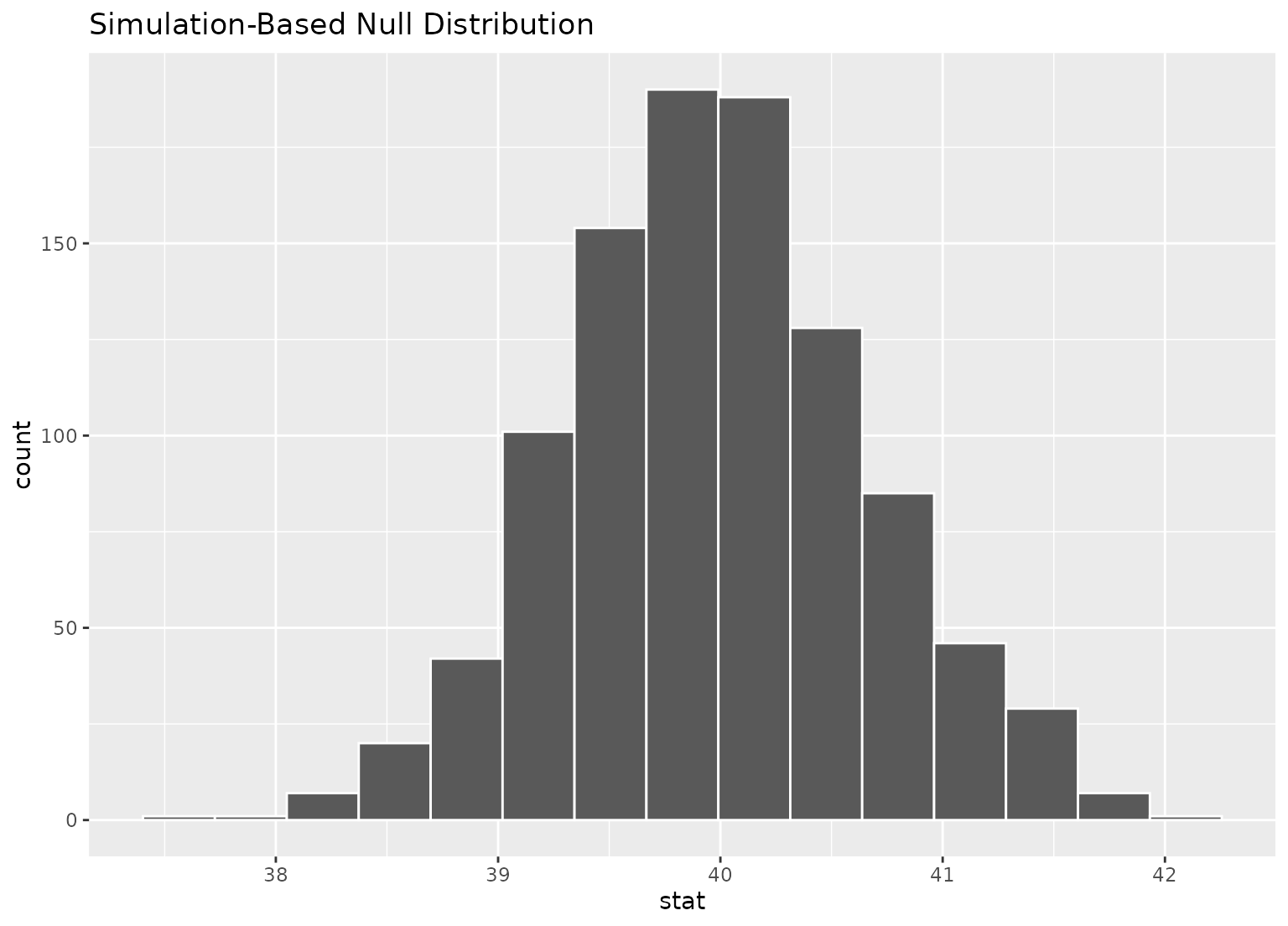# we can add layers to the plot as in ggplot, as well...
# find the point estimate---mean number of hours worked per week
point_estimate <- gss %>%
specify(response = hours) %>%
calculate(stat = "mean")

# find a confidence interval around the point estimate
ci <- boot_dist %>%
get_confidence_interval(point_estimate = point_estimate,
# at the 95% confidence level
level = .95,
# using the standard error method
type = "se")

# display a shading of the area beyond the p-value on the plot
null_dist %>%
visualize() +
shade_p_value(obs_stat = point_estimate, direction = "two-sided")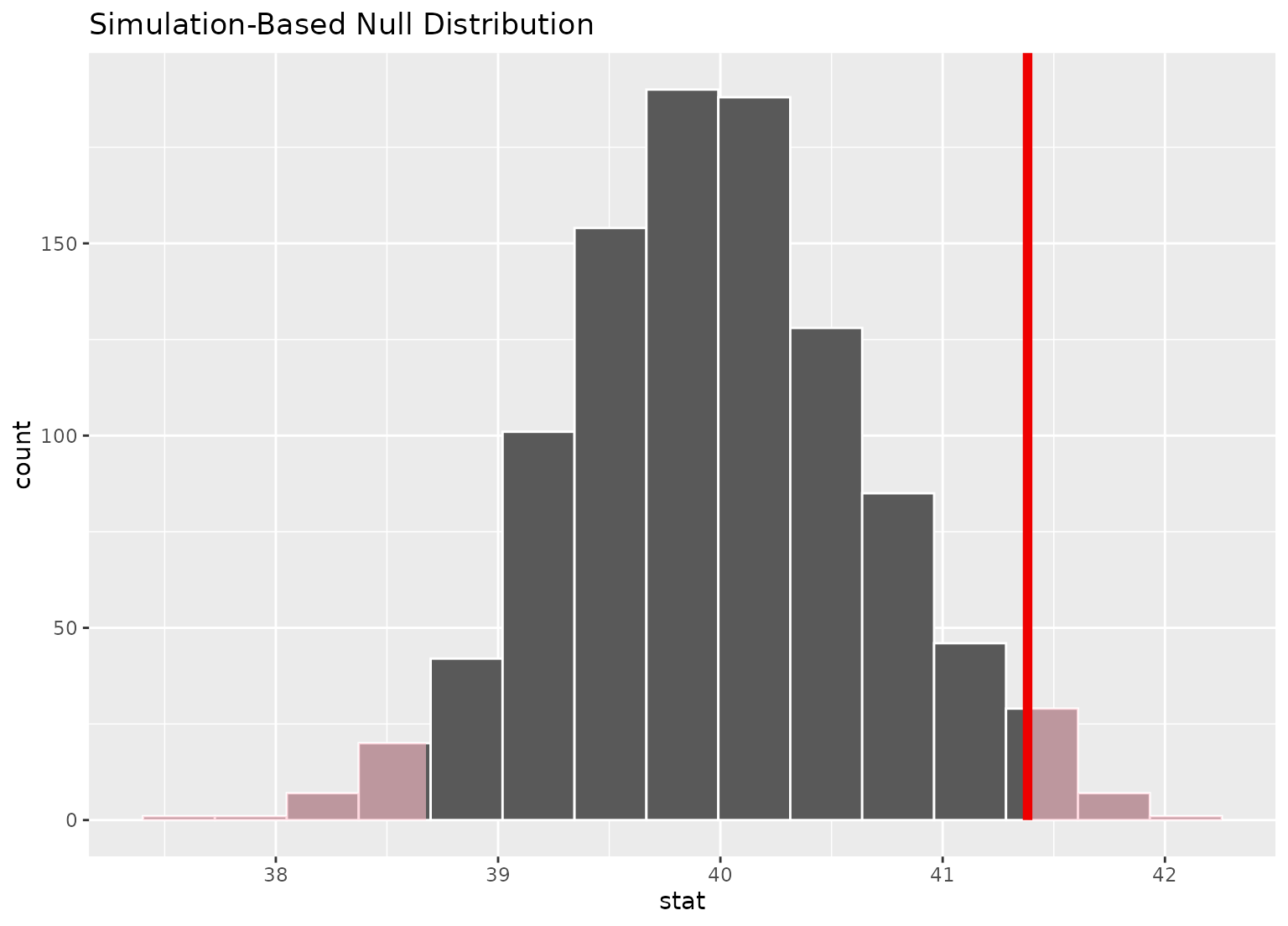# ...or within the bounds of the confidence interval
null_dist %>%
visualize() +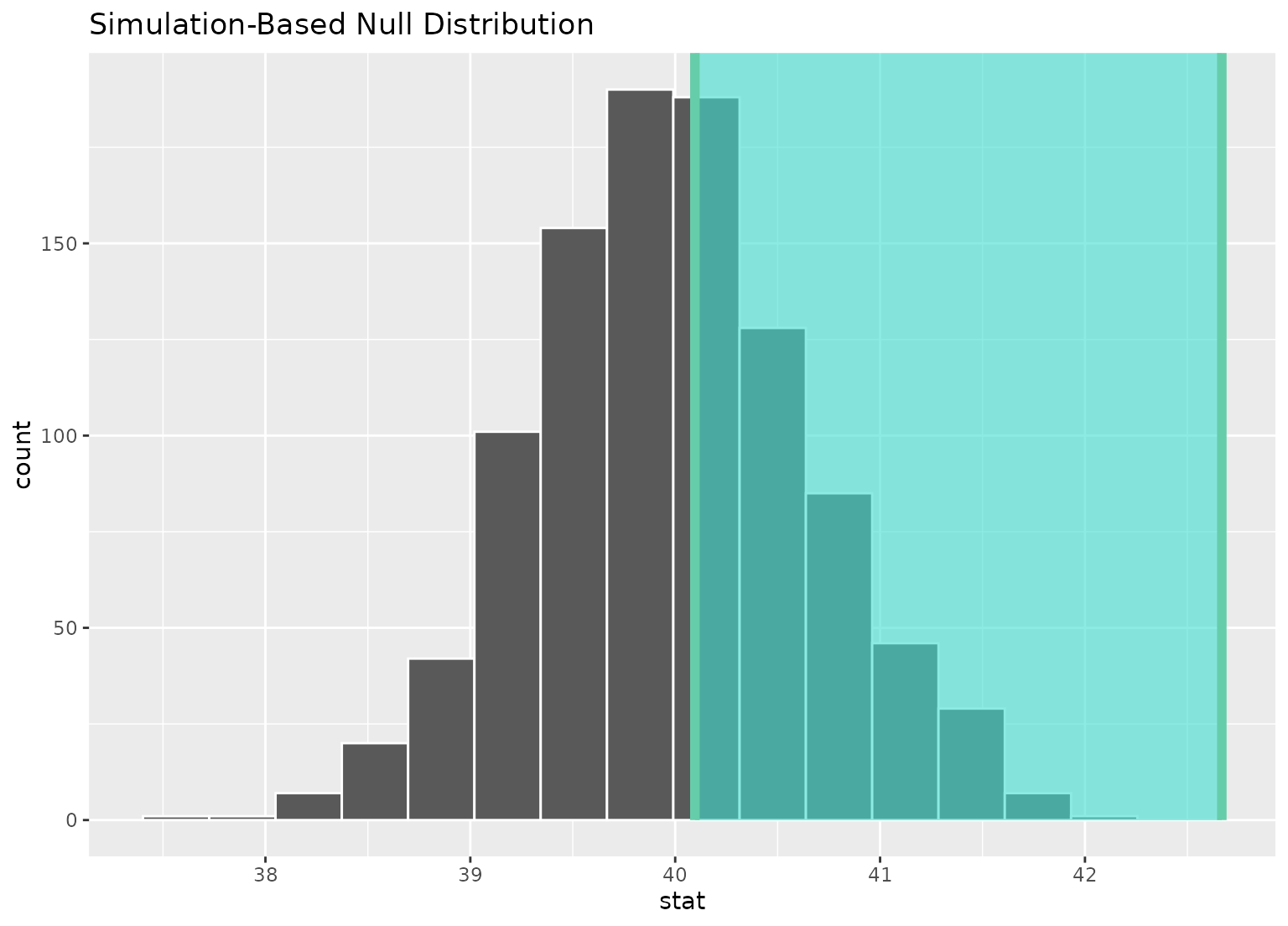# plot a theoretical sampling distribution by creating
# a theory-based distribution with assume()
sampling_dist <- gss %>%
specify(response = hours) %>%
assume(distribution = "t")

visualize(sampling_dist)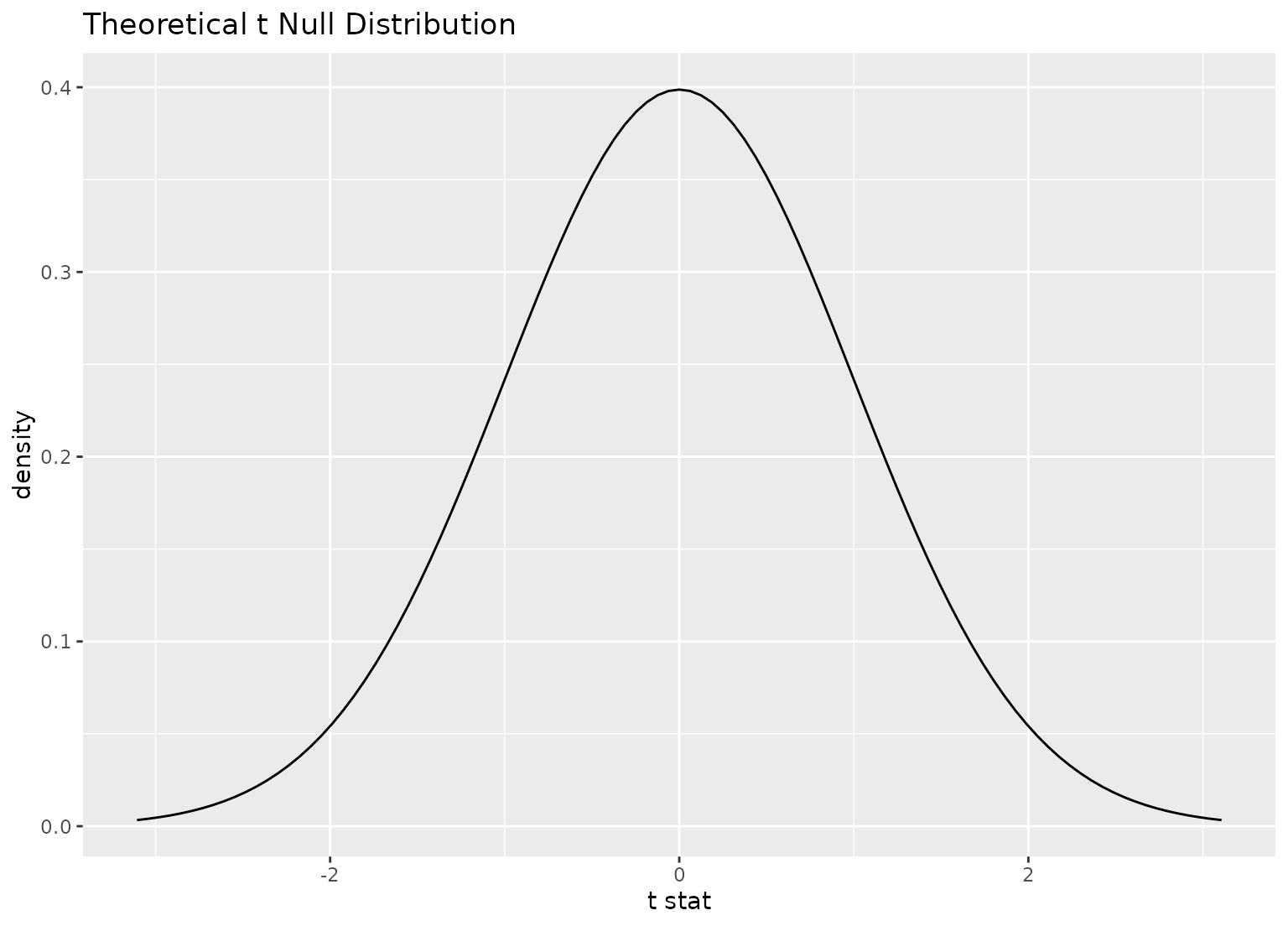# you can shade confidence intervals on top of
# theoretical distributions, too---the theoretical
# distribution will be recentered and rescaled to
# align with the confidence interval
visualize(sampling_dist) +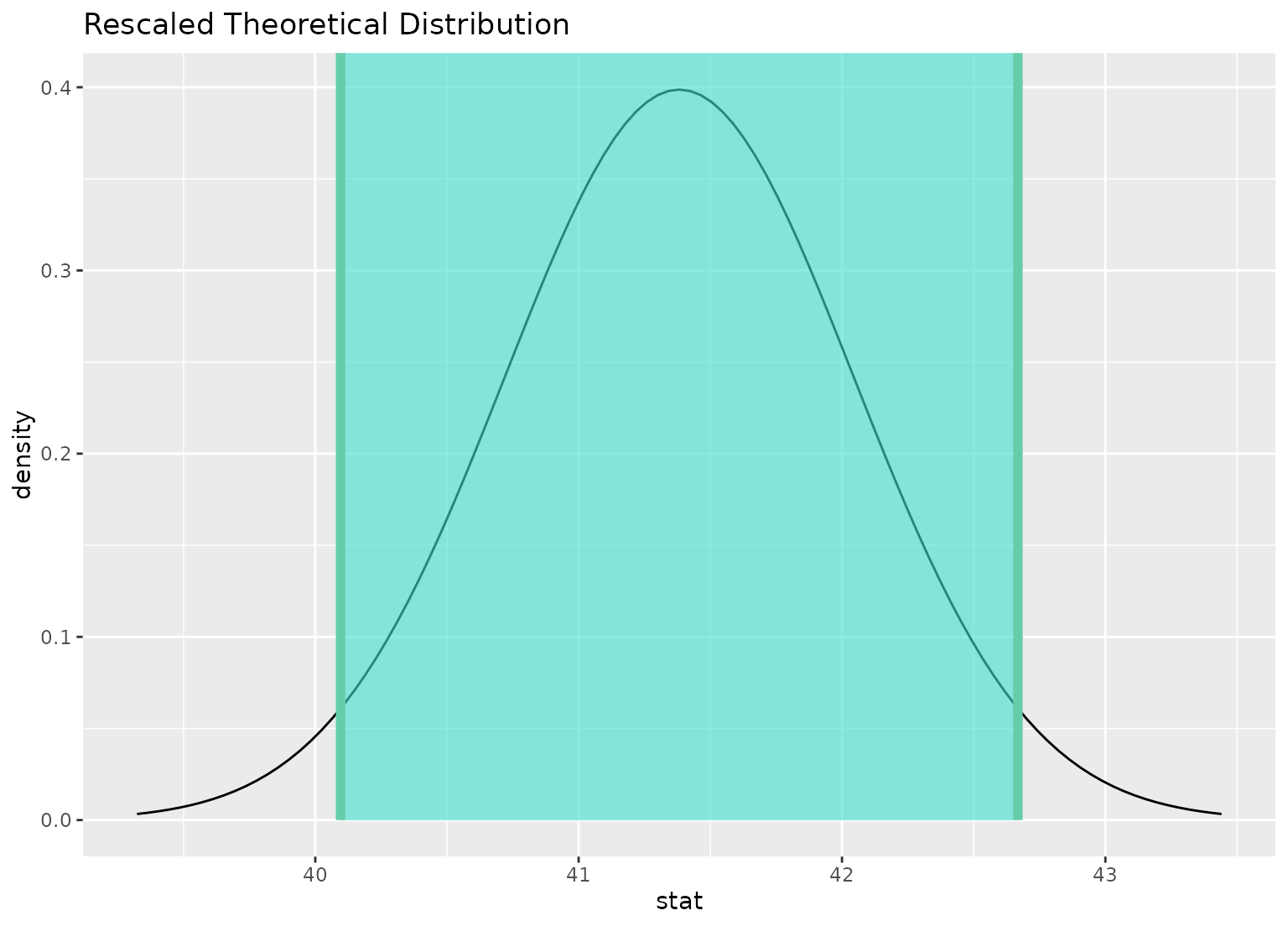# to plot both a theory-based and simulation-based null distribution,
# use a theorized statistic (i.e. one of t, z, F, or Chisq)
# and supply the simulation-based null distribution
null_dist_t <- gss %>%
specify(response = hours) %>%
hypothesize(null = "point", mu = 40) %>%
generate(reps = 1000, type = "bootstrap") %>%
calculate(stat = "t")

obs_stat <- gss %>%
specify(response = hours) %>%
hypothesize(null = "point", mu = 40) %>%
calculate(stat = "t")

visualize(null_dist_t, method = "both")
#> Warning: Check to make sure the conditions have been met for the theoretical method. {infer} currently does not check these for you.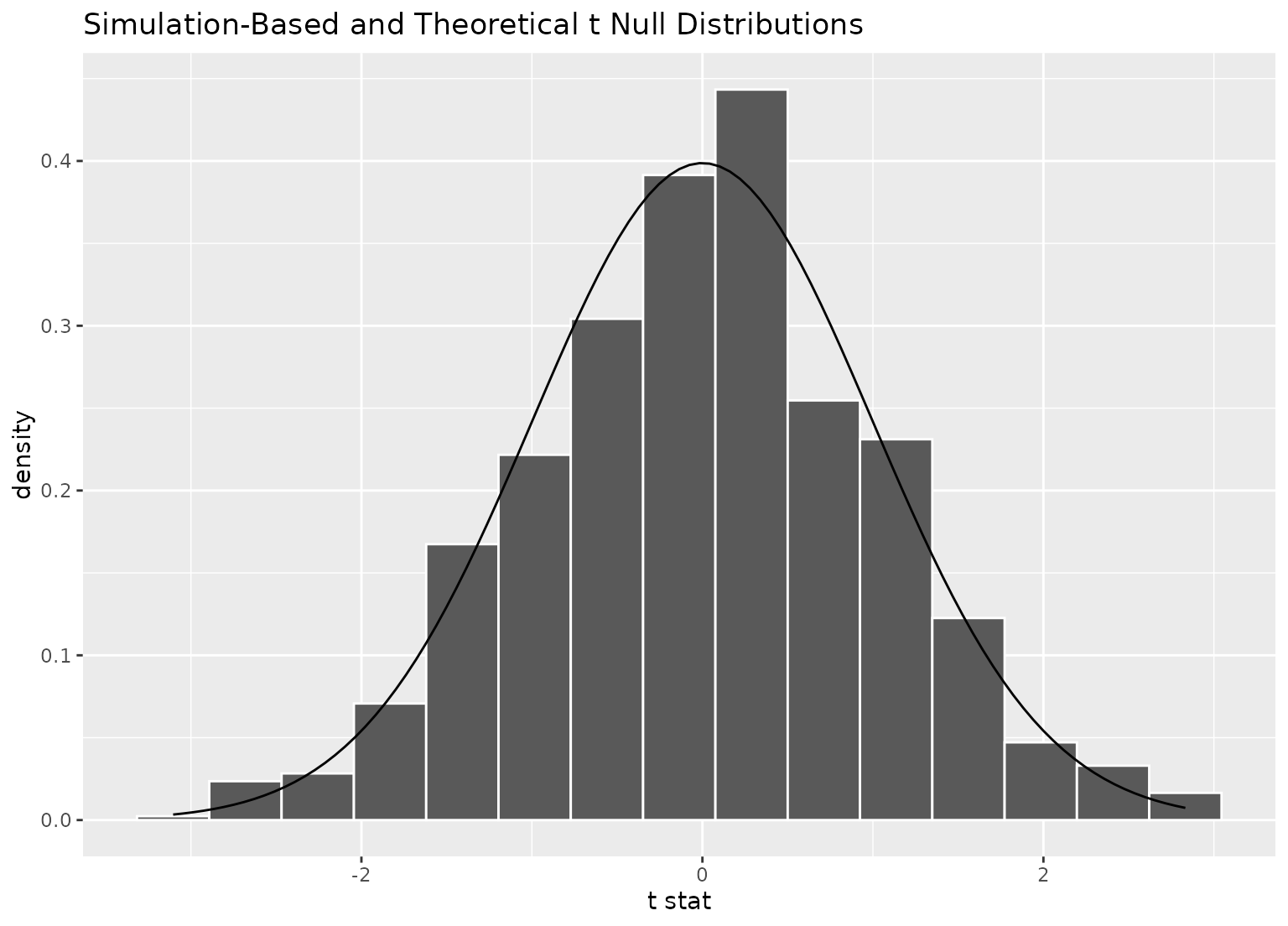visualize(null_dist_t, method = "both") +
#> Warning: Check to make sure the conditions have been met for the theoretical method. {infer} currently does not check these for you.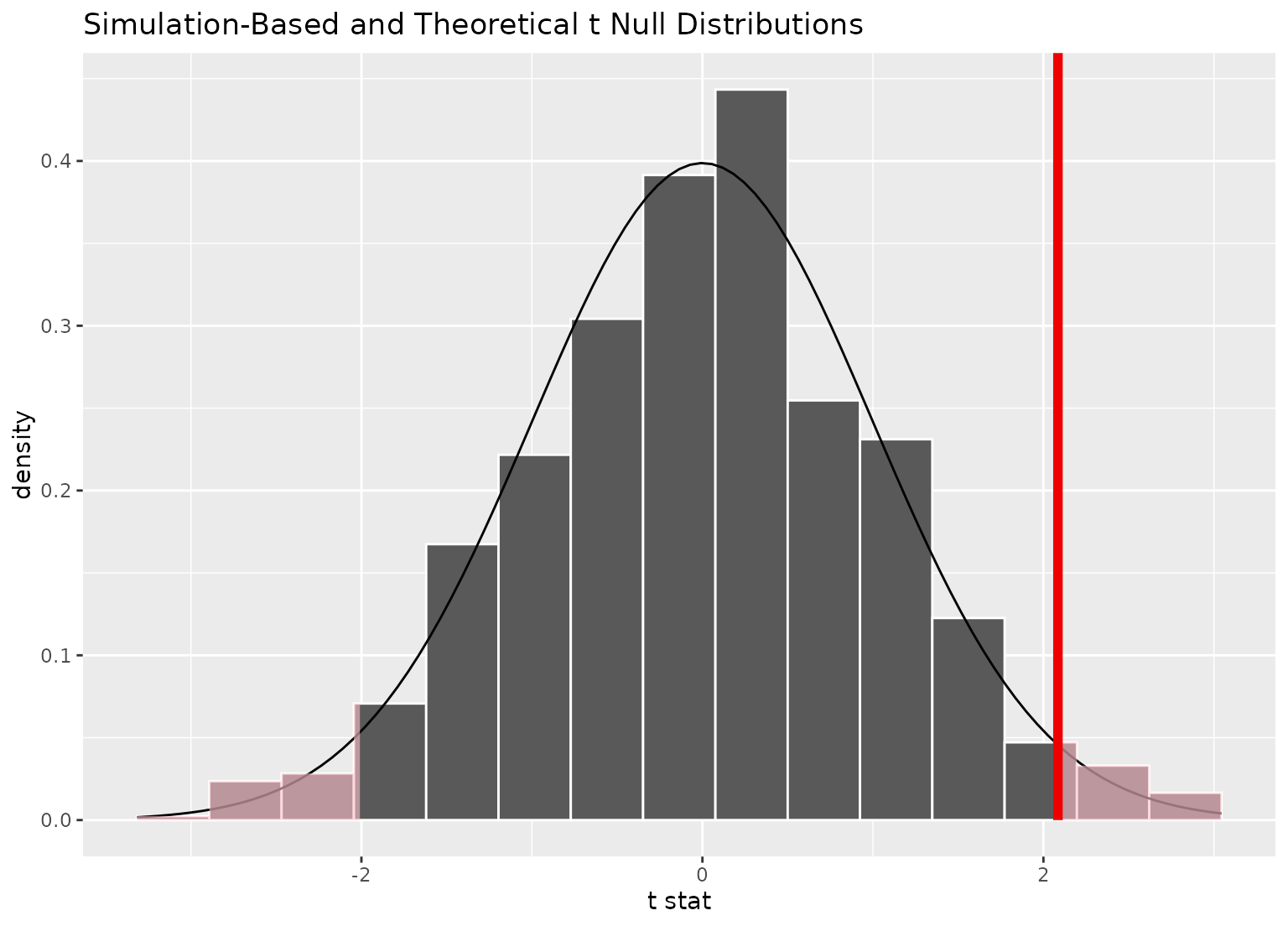# \donttest{
# to visualize distributions of coefficients for multiple
# explanatory variables, use a fit()-based workflow

# fit 1000 models with the hours variable permuted
null_fits <- gss %>%
specify(hours ~ age + college) %>%
hypothesize(null = "independence") %>%
generate(reps = 1000, type = "permute") %>%
fit()

null_fits
#> # A tibble: 3,000 × 3
#> # Groups:   replicate [1,000]
#>    replicate term          estimate
#>        <int> <chr>            <dbl>
#>  1         1 intercept      39.5
#>  2         1 age             0.0515
#>  3         1 collegedegree  -0.687
#>  4         2 intercept      40.5
#>  5         2 age             0.0209
#>  6         2 collegedegree  -0.0149
#>  7         3 intercept      39.8
#>  8         3 age             0.0305
#>  9         3 collegedegree   1.16
#> 10         4 intercept      39.9
#> # … with 2,990 more rows

# visualize distributions of resulting coefficients
visualize(null_fits)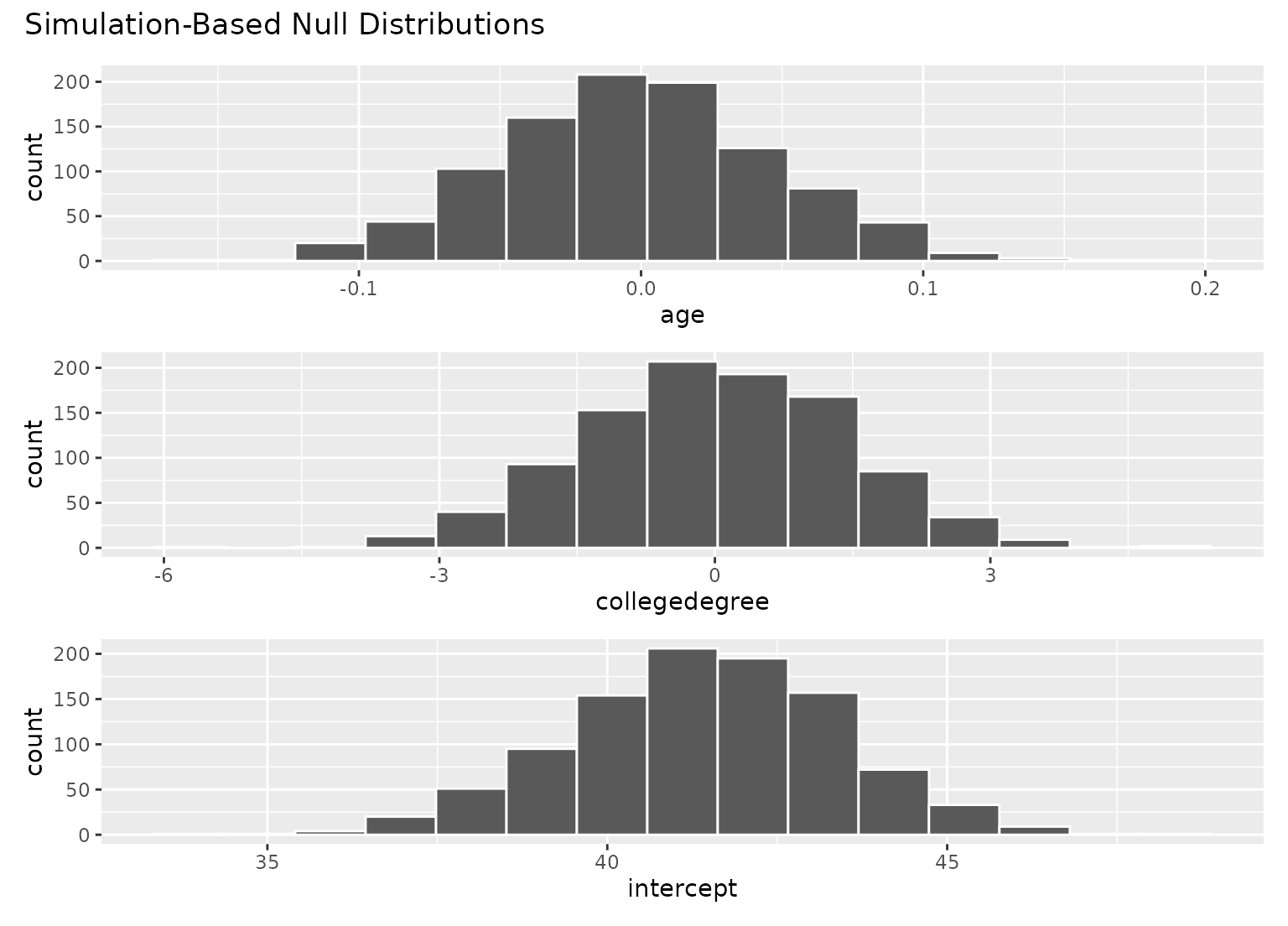# the interface to add themes and other elements to patchwork
# plots (outputted by visualize when the inputted data
# is from the fit() function) is a bit different than adding
# them to ggplot2 plots.
library(ggplot2)

# to add a ggplot2 theme to a calculate()-based visualization, use +
null_dist %>% visualize() + theme_dark()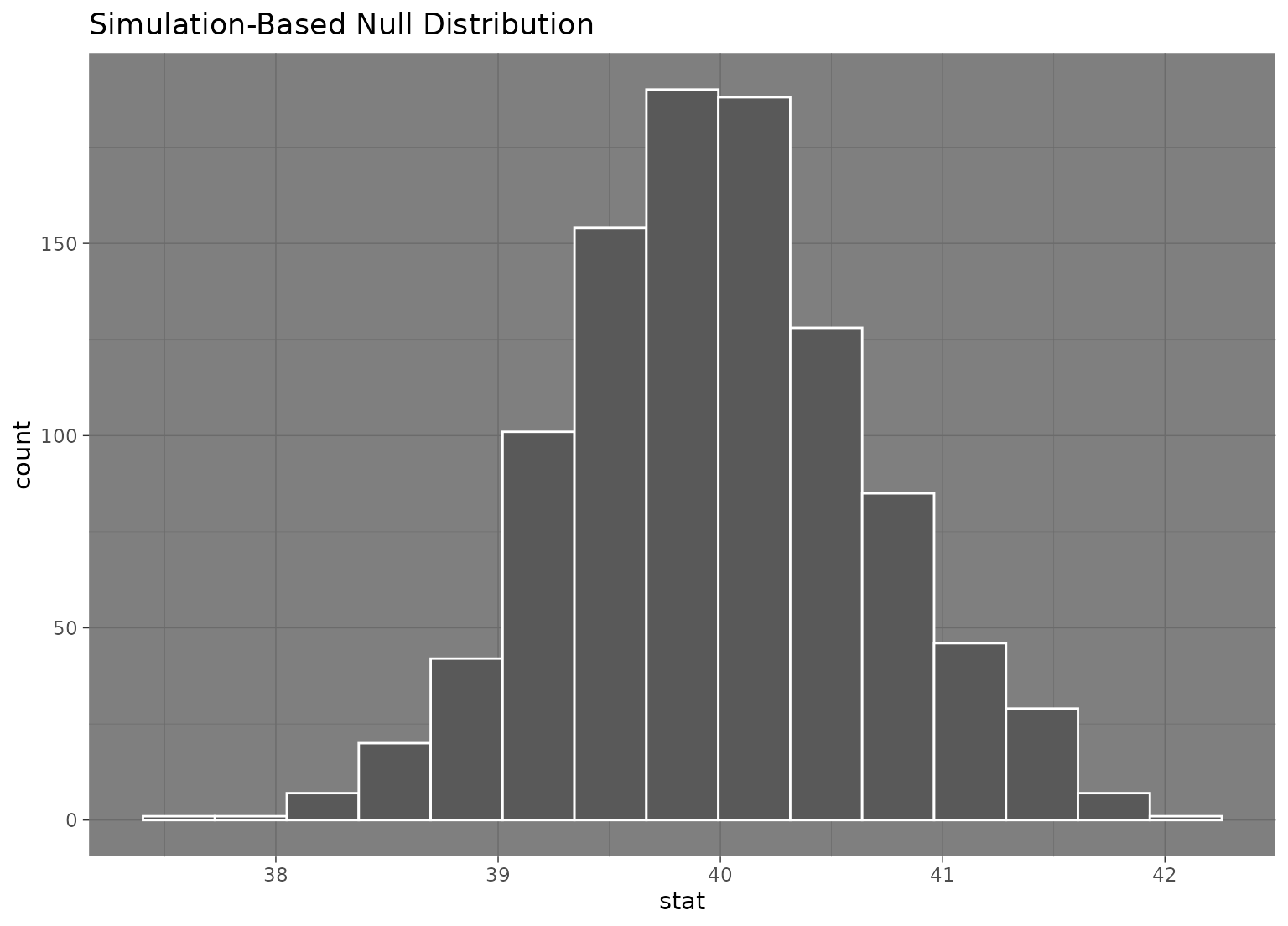# to add a ggplot2 theme to a fit()-based visualization, use &
null_fits %>% visualize() & theme_dark()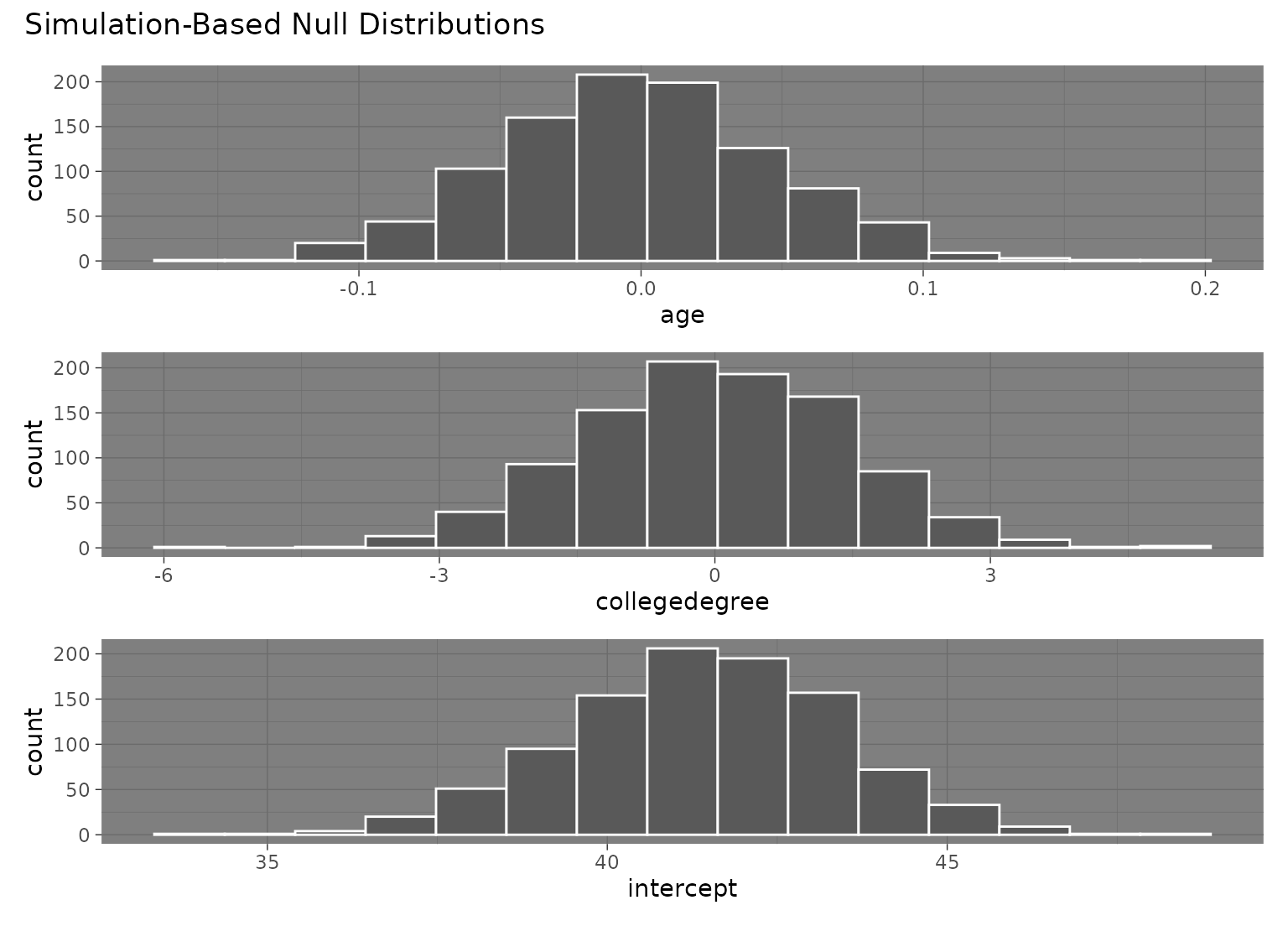# }

# More in-depth explanation of how to use the infer package
if (FALSE) {
vignette("infer")
}